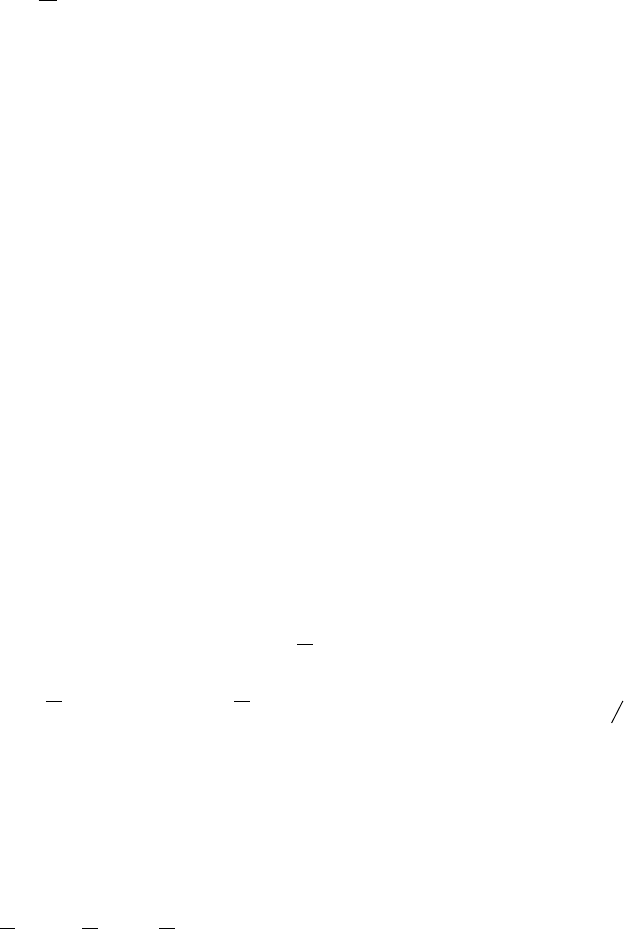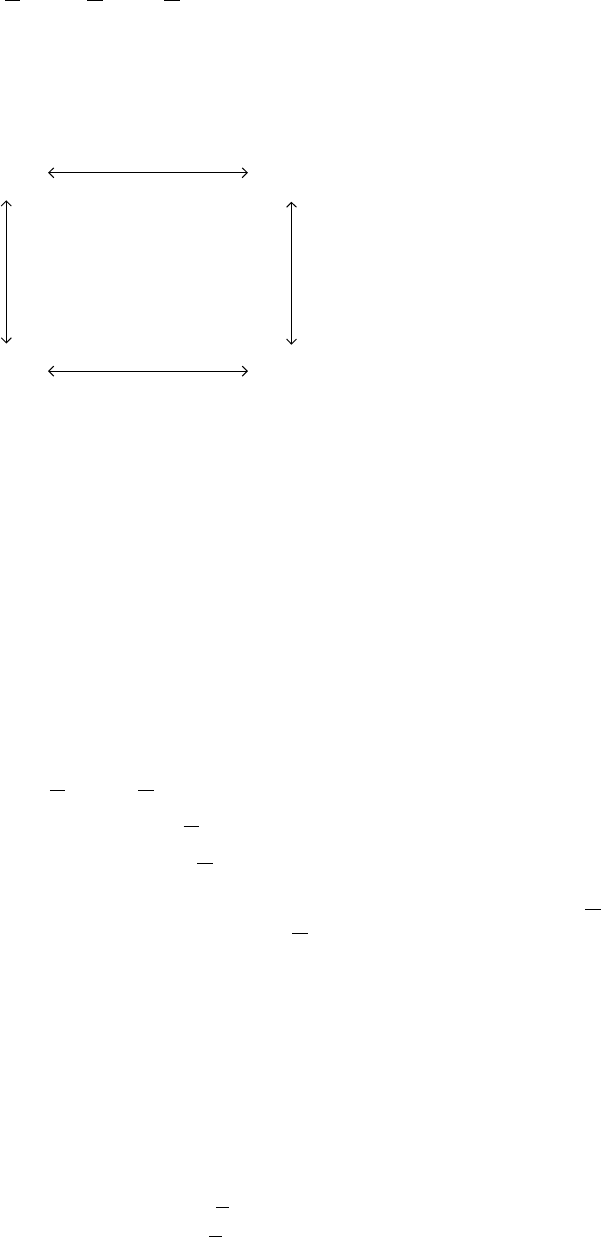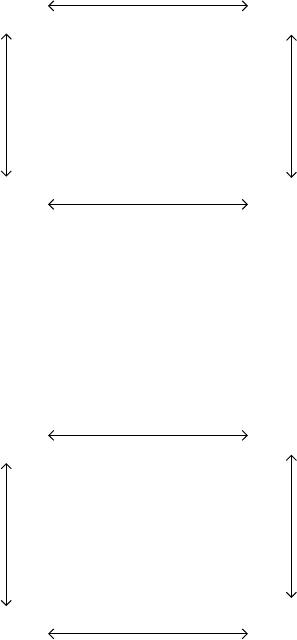Algebraic General Topology. Vol 1: Paperback / E-book || Axiomatic Theory of Formulas: Paperback / E-book

As an Amazon Associate I earn from qualifying purchases.Dual ﬁlters and ideals
by Victor Porton
Email: porton@narod.ru
Web: http://www.mathematics21.org
WARNING: There are errors in this draft. See instead my book.
[TODO: Deﬁne commutatitve diagrams.]
For a lattice Z I denote meets and joins correspondingly as u and t.
In my earlier work I denoted ﬁlters on a poset Z as F( Z) (or just F) and corresponding principal
ﬁlters as P(Z) (or just P).
I will denote X = A n X for a set X A.
Filters and ideals are well known concepts:
Filters F are subsets F of A such that:
1. F does not contain the least element of A (if it exists).
2. A u B 2 F , A 2 F ^ B 2 F (for every A; B 2 Z).
Ideals I are subsets F of A such that:
1. F does not contain the greatest element of A (if it exists).
2. A t B 2 F , A 2 F ^ B 2 F (for every A; B 2 Z).
Free stars S are subsets F of A such that:
1. F does not contain the least element of A (if it exists).
2. A t B 2 F , A 2 F _ B 2 F (for every A; B 2 Z).
Mixers M are subsets F of A such that:
1. F does not contain the greatest element of A (if it exists).
2. A u B 2 F , A 2 F _ B 2 F (for every A; B 2 Z).
Proposition 1. A set F is a lower set iﬀ F is an upper set.
Proof. X 2 F ^ Z w X ) Z 2 F is equivalent to Z 2 F ) X 2 F _ Z w X is equivalent
Z 2 F ) (Z w X ) X 2 F ) is equivalent Z 2 F ^ X v Z ) X 2 F .
I will denote dual A where A 2 Z the corresponding element of the dual poset Z
. Also I denote
hdualiX =
def
fdual x j x 2 X g:
Then we have the following bijections between above described four sets:
A t B 2 F , A 2 F _ B 2 F is equivalent to :(A t B 2 F ) , :(A 2 F ^ B 2 F ) is equivalent to
A t B 2 F , A 2 F ^ B 2 F ;
1A u B 2 F , A 2 F _ B 2 F is equivalent to :(A u B 2 F ) , :(A 2 F ^ B 2 F ) is equivalent to
A u B 2 F , A 2 F ^ B 2 F .
We have the following commutative diagram in category Set, every arrow of this diagram is an
isomorphism, every cycle in this diagram is an identity:
hduali
ideals
:
hduali
free stars
mixers
:
ﬁlters
Figure 1. Diagram Υ
(where : denotes set-theoretic complement).
These isomorphisms are also order isomorphisms if we deﬁne order in the right way.
The above it is deﬁned for lattices only. Generalizing this for arbitrary posets is straigthforward:
Deﬁnition 2. Let A be a poset.
Filters are sets F without the greatest element of A with A; B 2F ,9Z 2 F :(Z v A ^ Z v B)
(for every A; B 2 Z).
Ideals are sets F without the least element of A with A; B 2 F ,9Z 2 F : (Z w A ^ Z w B)
(for every A; B 2 Z).
Free stars are sets F without the greatest element of A with
A; B 2 F , 9Z 2 F : (Z w A ^ Z w B)
A 2/ F ^ B 2/ F , 9Z 2 F : (Z w A ^ Z w B)
A 2 F _ B 2 F , :9Z 2 F : (Z w A ^ Z w B)
Mixers are lower sets F without the least element of A with :9Z 2 F : (Z v A ^ Z v B) ,
A 2 F _ B 2 F or equivalently 9Z 2 F : (Z v A ^ Z v B) , A 2/ F ^ B 2/ F (for every A; B 2 Z).
Proposition 3. The following are equivelent: [TODO: With one side implications and requirement
to be upper/lower set.]
1. F is a free star.
2. 8Z 2 A: (Z w A ^ Z w B ) Z 2 F ) , A 2 F _ B 2 F for every A; B 2 A and F =/ P A.
Proof. The following is a chain of equivalencies:
9Z 2 F : (Z w A ^ Z w B) , A 2/ F ^ B 2/ F ;
8Z 2 F : :(Z w A ^ Z w B) , A 2 F _ B 2 F ;
8Z 2 A: (Z 2/ F ) :(Z w A ^ Z w B)) , A 2 F _ B 2 F ;
8Z 2 A: (Z w A ^ Z w B ) Z 2 F ) , A 2 F _ B 2 F :
2Corollary 4. The following are equivelent: [TODO: With one side implications and requirement
to be upper/lower set.]
1. F is a mixer.
2. 8Z 2 A: (Z v A ^ Z v B ) Z 2 F ) , A 2 F _ B 2 F for every A; B 2 A and F does not contain
the least element of A.
1 General isomorphisms
Let θ be an self-inverse order reversing isomorphism of some set P of posets.
We have the following commutative diagram in category Set, every arrow of this diagram is an
isomorphism, every cycle in this diagram is an identity:
hduali
ideals
:
hduali
free stars
mixers
:
F(P )
Figure 2. Diagram Υ
2 Boolean lattices
In the case if Z is a boolean lattice, there is also an alternative commutative diagram in category
Set, every arrow of this diagram is an isomorphism, every loop in this diagram is an identity:
h:i
ideals
:
h:i
free stars
mixers
:
ﬁlters
Figure 3. Diagram Ψ
(here h:iX =
def
fx¯ j x 2 X g).
[TODO: Other deﬁnition where upper/lower set is explicitly said.]
[TODO: Combine two rectangular diagrams into one “cubic”.]
Above we have two diagrams D with ??(every isomorphism). I will denote A!
D
B the unique
bijection from A to B which is a composition of arrows of this diagram.
[TODO: Deﬁne isomorphism of ﬁltrators.]
Deﬁne principal ideals, free stars, mixers as objects isomorphic to principal ﬁlters. [FIXME: There
are two diagrams which provide diﬀerent isomorphisms!] These isomorphisms f have in common
that fa v fb,a wb. We can deﬁne the isomorphism hduali
as a function such that fa v fb,a wb.
It is called order reversing isomorphism. So ﬁrst consider the general case of subsets of ﬁlters,
ideals, ... with an arbitrary antitone isomorphism. Need it to be an involution?
3